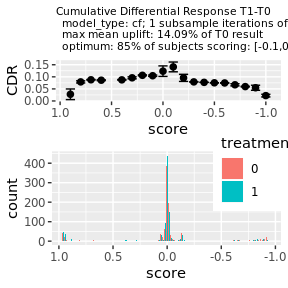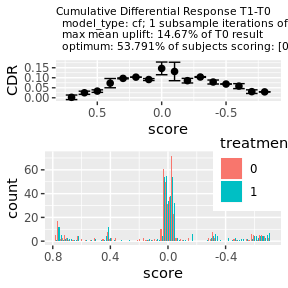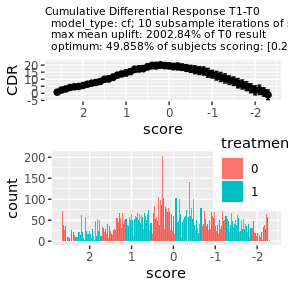# Setup

library(grf)
library(ggplot2)
library(uplifteval)

# Utility function round logistic function maps R -> {0,1}
rl <- function(x){
round(1/(1+exp(-x)))
}

# Generate feature data
set.seed(123)
n = 2000; p = 10
X = matrix(rnorm(n*p), n, p)
X.test = matrix(rnorm(n*p), n, p)

# Examples with outcomes in {0,1}

library(grf)
library(ggplot2)
library(uplifteval)

#
# Case 1: randomized control trial, treatment propensity is feature independent and equal
# for treatment and control cases, 50-50
#
# Treatment/Response Train/Test
set.seed(123)
W = rbinom(n, 1, 0.5)
W.test = rbinom(n, 1, 0.5)
Y = rl(rl(X[,1]) * W - rl(X[,3]) * W + rnorm(n))
Y.test = rl(rl(X.test[,1]) * W.test - rl(X.test[,3]) * W.test + rnorm(n))

tau.forest = causal_forest(X, Y, W)
tau.hat = predict(tau.forest, X.test)

plot_uplift(tau.hat$predictions, W.test, Y.test)# # Case 2: randomized control trial, treatment propensity is feature independent but unequal # for treatment and control cases, 80-20 # # Treatment/Response Train/Test set.seed(123) W = rbinom(n, 1, 0.8) W.test = rbinom(n, 1, 0.8) Y = rl(rl(X[,1]) * W - rl(X[,3]) * W + rnorm(n)) Y.test = rl(rl(X.test[,1]) * W.test - rl(X.test[,3]) * W.test + rnorm(n)) table(W.test, Y.test) ## Y.test ## W.test 0 1 ## 0 211 211 ## 1 782 796 rowSums(table(W.test, Y.test)) ## 0 1 ## 422 1578 tau.forest = causal_forest(X, Y, W) tau.hat = predict(tau.forest, X.test) plot_uplift(tau.hat$predictions, W.test, Y.test)# Examples with continuous outcomes

# Treatment/Response Train/Test
set.seed(123)
W = rbinom(n, 1, 0.8)
W.test = rbinom(n, 1, 0.8)
Y = X[,1] * W - X[,3] * W + rnorm(n)
Y.test = X.test[,1] * W.test - X.test[,3] * W.test + rnorm(n)

tau.forest = causal_forest(X, Y, W)
tau.hat = predict(tau.forest, X.test)

plot_uplift(tau.hat\$predictions, W.test, Y.test, n_bs = 10)